# JP2014211661A - Detection device, detection method, and detection program - Google Patents

## Info

Publication number
JP2014211661A
JP2014211661A JP2011185694A JP2011185694A JP2014211661A JP 2014211661 A JP2014211661 A JP 2014211661A JP 2011185694 A JP2011185694 A JP 2011185694A JP 2011185694 A JP2011185694 A JP 2011185694A JP 2014211661 A JP2014211661 A JP 2014211661A
Authority
JP
Japan
Prior art keywords
process
direction
data
θ
δθ
Prior art date
Legal status (The legal status is an assumption and is not a legal conclusion. Google has not performed a legal analysis and makes no representation as to the accuracy of the status listed.)
Withdrawn
Application number
JP2011185694A
Other languages
Japanese (ja)
Inventor

Osamu Nishida

Original Assignee
シャープ株式会社
Sharp Corp
Priority date (The priority date is an assumption and is not a legal conclusion. Google has not performed a legal analysis and makes no representation as to the accuracy of the date listed.)
Filing date
Publication date
Application filed by シャープ株式会社, Sharp Corp filed Critical シャープ株式会社
Priority to JP2011185694A priority Critical patent/JP2014211661A/en
Publication of JP2014211661A publication Critical patent/JP2014211661A/en
Application status is Withdrawn legal-status Critical

• 238000001514 detection method Methods 0 abstract title 2
• 238000000034 methods Methods 0 abstract 2
• 238000003860 storage Methods 0 abstract 2
• 230000035945 sensitivity Effects 0 abstract 1

## Images

•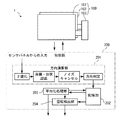••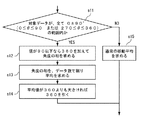•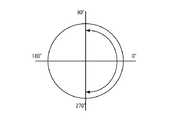•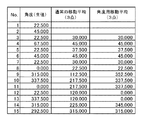••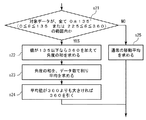•••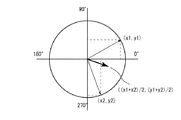•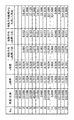## Classifications

• GPHYSICS
• G06COMPUTING; CALCULATING; COUNTING
• G06FELECTRIC DIGITAL DATA PROCESSING
• G06F3/00Input arrangements for transferring data to be processed into a form capable of being handled by the computer; Output arrangements for transferring data from processing unit to output unit, e.g. interface arrangements
• G06F3/01Input arrangements or combined input and output arrangements for interaction between user and computer
• G06F3/03Arrangements for converting the position or the displacement of a member into a coded form
• G06F3/041Digitisers, e.g. for touch screens or touch pads, characterised by the transducing means
• G06F3/0416Control or interface arrangements specially adapted for digitisers
• GPHYSICS
• G06COMPUTING; CALCULATING; COUNTING
• G06KRECOGNITION OF DATA; PRESENTATION OF DATA; RECORD CARRIERS; HANDLING RECORD CARRIERS
• G06K9/00Methods or arrangements for reading or recognising printed or written characters or for recognising patterns, e.g. fingerprints
• G06K9/00362Recognising human body or animal bodies, e.g. vehicle occupant, pedestrian; Recognising body parts, e.g. hand
• G06K9/00375Recognition of hand or arm, e.g. static hand biometric or posture recognition
• GPHYSICS
• G06COMPUTING; CALCULATING; COUNTING
• G06TIMAGE DATA PROCESSING OR GENERATION, IN GENERAL
• G06T7/00Image analysis
• G06T7/70Determining position or orientation of objects or cameras
• G06T7/73Determining position or orientation of objects or cameras using feature-based methods
• G06T7/74Determining position or orientation of objects or cameras using feature-based methods involving reference images or patches

## Abstract

Even when the sensitivity of a sensor is not sufficient, the direction of the object is stably obtained from a two-dimensional image of an object that is in contact with or close to the sensor (for example, an input device such as a finger or a pen, or an article to be observed). A detection device, a detection method, and a detection program are obtained.
A detection device 1 is capable of recognizing a two-dimensional image of an object that is in contact with or close to it, and outputs direction data representing the direction of the object from the two-dimensional image recognized by the sensor unit 101. A direction calculation unit 201, a storage unit 202 that continuously samples and stores the direction data output from the direction calculation unit 201, and an average that performs an averaging process based on the direction data stored in the storage unit 202 The processing unit 203 is provided.
[Selection] Figure 1

## Description

The present invention relates to a detection device, a detection method, and a detection program, and more particularly to a detection device, a detection method, and a detection program that detect the direction of an object that is in contact with or close to a sensor.

In recent years, devices equipped with a detection device typified by a touch panel are rapidly spreading. The functions of these devices are diversified and complicated, and accordingly, more various operation methods are required.

Patent Document 1 describes a touch panel type input device that determines the direction of a user's hand from the shape of a finger on the touch panel.

JP 2008-52536 A

However, when the sensitivity of the sensor (touch panel) is particularly low, accurate recognition may not be possible depending on the input data (finger shape). In such a case, the determined direction becomes inaccurate or fluctuates.

The object of the present invention is to stabilize the direction of an object from a two-dimensional image of an object (for example, an input device such as a finger or a pen, an object to be observed) that is in contact with or close to the sensor even when the sensitivity of the sensor is not sufficient. And obtaining a detection device, a detection method, and a detection program.

The detection device disclosed below includes a sensor unit capable of recognizing a two-dimensional image of an object that is in contact with or in proximity, and a direction calculation that outputs direction data representing the direction of the object from the two-dimensional image recognized by the sensor unit. A storage unit that continuously samples and stores the direction data output from the direction calculation unit, and an averaging processing unit that performs an averaging process based on the direction data stored in the storage unit.

In addition, the detection method disclosed below outputs a direction calculation process that outputs direction data representing the direction of an object in the data from two-dimensional image data, and continuously samples and stores the result of the direction calculation process. Storage processing, and averaging processing performed based on the data stored by the storage processing.

In addition, the computer program disclosed below outputs a direction calculation process for outputting direction data representing the direction of an object in the data from two-dimensional image data, and continuously samples and stores the result of the direction calculation process. Storage processing to be performed, and averaging processing to be performed based on the data stored by the storage processing.

Even when the sensitivity of the sensor is not sufficient, the direction of the object can be stably obtained from a two-dimensional image of an object that is in contact with or close to the sensor (for example, an input device such as a finger or a pen, or an article to be observed). , A detection apparatus, a detection method, and a detection program can be obtained.

FIG. 1 is a block diagram schematically showing the configuration of the detection apparatus according to the first embodiment of the present invention. FIG. 2 is a diagram for explaining an example of a method for determining the direction of a finger or the like in the first embodiment of the present invention. FIG. 3A is a flowchart illustrating an angle averaging process of the detection device according to the first embodiment of the present invention. FIG. 3B is a diagram for explaining an angle averaging process of the detection device according to the first embodiment of the present invention. FIG. 4 is a table comparing a normal moving average and an angle averaging process according to the first embodiment of the present invention with specific examples. FIG. 5 is a diagram comparing a normal moving average and a value obtained by an angle averaging process according to an embodiment of the present invention. FIG. 6A is a flowchart illustrating an angle averaging process of the detection device according to the second embodiment of the present invention. FIG. 6B is a diagram for explaining an angle averaging process of the detection device according to the second embodiment of the present invention. FIG. 7A is a flowchart illustrating an angle averaging process of the detection device according to the third embodiment of the present invention. FIG. 7B is a diagram for explaining an angle averaging process of the detection device according to the third embodiment of the present invention. FIG. 8 is a table showing a specific example of the angle averaging process according to the third embodiment of the present invention.

A detection apparatus according to an embodiment of the present invention includes a sensor unit capable of recognizing a two-dimensional image of an object that is in contact with or in close proximity, and direction data representing the direction of the object from the two-dimensional image recognized by the sensor unit. An output direction calculation unit, a storage unit that continuously samples and stores the direction data output by the direction calculation unit, and an averaging processing unit that performs an averaging process based on the direction data stored in the storage unit (A first configuration of the detection device).

According to said structure, a memory | storage part samples and memorize | stores direction data continuously. Then, the averaging processing unit performs an averaging process based on the direction data stored in the storage unit. Thereby, the influence of inaccurate direction data and fluctuation of the direction data is alleviated, and stable direction data can be obtained.

Note that this “averaging process” does not only refer to the process of calculating the average (arithmetic mean) of the sum of each data divided by the number of data points, but instead of the weighted average, Includes processing for obtaining harmonic averages and the like.

In the first configuration of the detection device, the storage unit outputs the direction data output from the direction calculation unit as angle data that takes a value not less than θ 0 and less than θ 1 that satisfies the relationship θ 1 −θ 0 = 360. The averaging processing unit stores Δθ 0 and Δθ 1 as predetermined numbers, and when all of the angle data to be averaged are not more than Δθ 0 and less than Δθ 1 , Δθ 0 The following processing can be performed including processing for adding 360 to the angle data, processing for obtaining an average, and processing for subtracting θ 1 when the obtained average is equal to or greater than θ 1 (the first detection device). 2 configuration).

According to said structure, a memory | storage part memorize | stores direction data as angle data which takes the value more than (theta) 0 and less than (theta) 1 which satisfy | fill the relationship of (theta) 1- (theta) 0 = 360. Thereby, a memory | storage part memorize | stores direction data as angle data defined uniquely. Further, when all the angle data to be averaged are not more than Δθ 0 and less than Δθ 1 , the averaging processing unit adds 360 ° to the angle data equal to or less than Δθ 0 and obtains the average. . This avoids the problem of circulating angle values. Then, averaging processing unit, the average obtained pulls the theta 1 when one or more theta. As a result, the angle is corrected to a uniquely defined range.

Here, the angle stored in the storage unit is expressed by the frequency method, but the present invention can be applied regardless of the angle notation method. For example, when the angle is expressed by the arc method, 360 described above may be replaced with 2π.

In the first configuration of the detection device, the storage unit stores the direction data output from the direction calculation unit as angle data, and the averaging processing unit stores the angle data stored in the storage unit as a circle. It can be configured to perform processing including processing for converting to coordinates on the circumference, processing for obtaining an average based on the converted coordinates, and processing for converting the averaged coordinates into angle data (detection) Third configuration of the device).

The angle can be averaged also by the above configuration.

In any one of the first to third configurations of the detection device, a configuration may further include a rotation detection unit that detects a rotation operation based on a change in the averaged direction data calculated by the averaging processing unit. Good (4th structure of a detection apparatus).

According to said structure, a rotation detection part detects rotation operation | movement based on the change of direction data. 2. Description of the Related Art Conventionally, an input method for inputting a rotation operation by rotating two fingers on a touch panel is known. According to the 4th structure of the detection apparatus of this invention, the input method which inputs rotation operation with one finger | toe is realizable by determining the direction of the finger | toe on a touch panel, for example.

The rotation detection unit detects a rotation operation based on a change in the averaged direction data calculated by the averaging processing unit. As a result, inaccurate direction data and erroneous detection due to fluctuations in the direction data can be suppressed.

A detection method according to an embodiment of the present invention includes: a direction calculation process that outputs direction data representing the direction of an object in the data from two-dimensional image data; and a result of the direction calculation process is continuously sampled. Storage processing, and averaging processing performed based on the data stored by the storage processing (first configuration of the detection method).

In the first configuration of the detection method, the storage process uses the direction data calculated by the direction calculation process as angle data that takes a value not less than θ 0 and less than θ 1 that satisfies the relationship θ 1 −θ 0 = 360. The averaging process stores Δθ 0 and Δθ 1 as predetermined numbers, and when all the angle data to be averaged are not greater than Δθ 0 and less than Δθ 1 , Δθ 0 or less. A process including adding 360 to the angle data, a process of obtaining an average, and a process of subtracting θ 1 when the obtained average is equal to or greater than θ 1 can be employed (second configuration of the detection method).

In the first configuration of the detection method, the storage process stores the direction data calculated by the direction calculation process as angle data, and the averaging process uses the direction data stored by the storage process as a circle. It can be configured to include a process for converting to a coordinate on the circumference, a process for obtaining an average based on the converted coordinate, and a process for converting the averaged coordinate to angle data (first detection method) 3 configuration).

In any one of the first to third configurations of the detection method, a configuration may further include a rotation detection process that detects a rotation operation based on a change in the averaged direction data calculated by the averaging process. Good (fourth configuration of detection method).

A computer program according to an embodiment of the present invention continuously samples direction calculation processing for outputting direction data representing the direction of an object in the data from two-dimensional image data, and results of the direction calculation processing. The computer executes the storage process to be stored and the averaging process based on the data stored by the storage process (first configuration of the computer program).

In the first configuration of the computer program, the storage process uses the direction data calculated by the direction calculation process as angle data that takes a value not less than θ 0 and less than θ 1 that satisfies the relationship θ 1 −θ 0 = 360. The averaging process stores Δθ 0 and Δθ 1 as predetermined numbers, and when all the angle data to be averaged are not greater than Δθ 0 and less than Δθ 1 , Δθ 0 or less. The processing may include a process of adding 360 to the angle data, a process of obtaining an average, and a process of subtracting θ 1 when the obtained average is equal to or greater than θ 1 (second configuration of the computer program).

In the first configuration of the computer program, the storage process stores the direction data calculated by the direction calculation process as angle data, and the averaging process uses the direction data stored by the storage process as a circle. A process for converting the coordinates into circumference coordinates, a process for obtaining an average based on the converted coordinates, and a process for converting the averaged coordinates into angle data (first computer program) 3 configuration).

In any one of the first to third configurations of the computer program, the computer further executes a rotation detection process for detecting a rotation operation based on a change in the averaged direction data calculated by the averaging process. It can be configured (fourth configuration of a computer program).

[Embodiment]
Hereinafter, embodiments of the present invention will be described in detail with reference to the drawings. In the drawings, the same or corresponding parts are denoted by the same reference numerals and description thereof will not be repeated.

[First Embodiment]
[Overall configuration]
FIG. 1 is a block diagram schematically showing a configuration of a detection apparatus 1 according to an embodiment of the present invention. The detection device 1 includes a sensor panel 100 and a control unit 200.

The sensor panel 100 includes a sensor unit 101, a display device 102, and a drive circuit 103. The display device 102 is not an essential component. That is, the sensor panel 100 may be configured to include the sensor unit 101 and the drive circuit 103.

The sensor unit 101 is, for example, a touch panel, a track pad, or a digitizer. The method of the sensor unit 101 is not particularly limited. When the sensor unit 101 is a touch panel, a capacitance type, a resistance film type, an optical type, or the like can be employed. On the other hand, the sensor unit 101 needs to be able to recognize an object in contact with or close to the sensor unit 101 as a two-dimensional image. For this reason, a projection type is preferable if it is a capacitive touch panel, and a matrix resistance film is preferable if it is a resistance film type touch panel. Further, the higher the resolution of the sensor unit 101, the better.

The display device 102 is, for example, a liquid crystal display device. The display device 102 may also serve as the sensor unit 101. For example, a display device with a built-in touch panel in which a photodiode or the like is incorporated in a pixel of the display device 102 may be adopted as the display device 102.

The drive circuit 103 is connected to the sensor unit 101 and the display device 102 via an FPC (Flexible Printed Circuit) or the like. The drive circuit 103 drives the sensor unit 101 and the display device 102.

The control unit 200 inputs a signal from the sensor panel 100. Further, a part of the signal is fed back to the sensor panel 100 as necessary. The control unit 200 analyzes the data of the two-dimensional image included in the input signal from the sensor panel 100, and calculates the position where the object is in contact with or close to the sensor unit 101 and the direction data of the object. Then, the calculated result is output to the outside. The control unit 200 may be mounted as a module on the drive circuit 103, or may be mounted on a device separate from the sensor panel 100. Further, not all of the control unit 200 may be implemented as hardware. That is, a part of the control unit 200 may be a virtual functional block that is realized by a processor executing a computer program.

The control unit 200 includes a direction calculation unit 201, a storage unit 202, and an averaging processing unit 203.

The direction calculation unit 201 continuously samples the two-dimensional image signal from the sensor panel 100. Then, the direction calculation unit 201 analyzes the sampled two-dimensional image signal and determines the direction of the object that is in contact with or close to the sensor unit 101.

The direction calculation unit 201 first binarizes a two-dimensional image signal input from the sensor panel 100. Based on the binarized data, the coordinates of the object on the sensor unit 101 and the shape of the object are calculated.

Thereafter, noise cancellation processing is performed. Specifically, processing for removing signals that are equal to or lower than a predetermined threshold value, processing for combining adjacent points within a certain range, and the like are performed. Then, the direction of the object is determined based on the noise-cancelled shape.

The method for determining the direction is arbitrary and is not particularly limited. An example of a method for determining the direction will be described with reference to FIG. In this example, first, the center of gravity P of the finger shape f adjacent to the sensor unit 101 is obtained. Then, a point S that is farthest from the center of gravity P on the contour of the shape f is obtained. A vector connecting P and S is a finger direction.

The calculation result of the direction calculation unit 201 is output to the storage unit 202. The storage unit 202 continuously samples and stores the direction data output from the direction calculation unit 201.

Based on the direction data stored in the storage unit 202, the averaging processing unit 203 performs an averaging process for reducing blurring of the direction data. Specifically, for example, the average is obtained by using the latest and latest direction data stored in the storage unit 202.

Note that this “averaging process” does not only refer to the process of calculating the average (arithmetic mean) of the sum of each data divided by the number of data points, but instead of the weighted average, Includes processing for obtaining harmonic averages and the like. Moreover, you may perform the process which calculates | requires a median and a mode value. Alternatively, an average may be obtained after removing a value greatly deviating from the median or mode.

The direction data averaged by the averaging processing unit 203 is output to the outside. The output of the averaging processing unit 203 is also output to the storage unit 202 as necessary.

The control unit 200 may further include a rotation detection unit 204. The rotation detection unit 204 detects a change in the direction data as a rotation operation based on the direction data output from the averaging processing unit 203 and the direction data stored in the storage unit 202. Then, data such as rotation angle, angular velocity, and acceleration are output to the outside.

The overall configuration of the detection device 1 according to one embodiment of the present invention has been described above. According to the present embodiment, the averaging process is performed based on values continuously sampled and stored in the storage unit. By this averaging process, the direction of the object can be stably obtained even when the sensitivity of the sensor unit 101 is insufficient.

[Angle averaging]
Hereinafter, a case where the direction data stored in the storage unit 202 is angle data represented by 0 ° ≦ θ <360 ° will be described. The method for defining the angle is arbitrary. For example, an angle θ represented by −180 ° ≦ θ <180 ° may be output and stored. Alternatively, the angle θ expressed using the arc degree method instead of the frequency method may be output and stored.

The angle circulates in value (turns around 360 °). For this reason, an average cannot be taken as it is. For example, the average value of 1 ° and 359 ° is 180 °. On the other hand, if 359 ° is expressed as −1 °, the average value is 0 °.

Hereinafter, an example of the angle averaging process will be described in detail.

FIG. 3A is a flowchart in the case of obtaining an average (moving average) using the latest data stored in the storage unit 202 and a plurality of times from the latest data. The number of data to be averaged is arbitrary.

First, in step s11, it is checked whether all the target angle data are within a certain range from 0 ° as the starting point of circulation. Specifically, it is examined whether all the angle data is in the range of 0 ° ± 90 °, that is, in the range of 0 ° ≦ θ ≦ 90 ° or 270 ° ≦ θ ≦ 360 ° (see FIG. 3B). In other words, it is checked that all the target angle data are not in the range of more than 90 ° and less than 270 °. And when satisfy | filling this condition, the special process after step s12 is performed. On the other hand, if this condition is not satisfied, the process proceeds to step s15 to obtain a normal moving average.

If the above condition is satisfied, 360 ° is added to the angle data of 90 ° or less in step s12. By this operation, the data that existed at 0 ° ≦ θ ≦ 90 ° and 270 ° ≦ θ ≦ 360 ° are present in a continuous range of 270 ° ≦ θ ≦ 450 °. After this operation, the sum is obtained for all angles.

In step 13, the average is obtained by dividing the sum of angles by the number of data. In step 14, if the average value is 360 ° or more, 360 ° is subtracted so that the value falls within the range of 0 ° ≦ θ <360 °.

FIG. 4 is a table comparing a normal average and an angle averaging process according to the present embodiment with specific examples. The “angle (raw value)” column shows the angle data output by the direction calculation unit 201 or the angle data stored in the storage unit 202. The column of “ordinary moving average (3 points)” shows the average value of the angle data of the three most recent points. The column of “Moving average for angles (3 points)” shows values obtained by performing an angle averaging process according to the present embodiment based on the angle data of the latest three points.

For example, in the table of FIG. If the angles of 8, 9, 10 are simply averaged, an angle of (0 + 315 + 337.5) /3=217.5 is obtained. On the other hand, when the angle averaging process according to the present embodiment is performed, an angle of ((0 + 360) + 315 + 337.5) /3=337.5 is obtained. As is apparent from FIG. 5, 337.5 is a value that better represents the angles (0, 315, 337.5) of these three points than 217.5.

According to this embodiment, the angle averaging process can be performed.

[Second Embodiment]
The second embodiment of the present invention differs from the first embodiment in the angle averaging process.

FIG. 6A is a flowchart in the case where the moving average is obtained using the latest data stored in the storage unit 202 and a plurality of angle data from the latest data. The number of angle data for taking a moving average is arbitrary.

First, in step s21, it is checked whether all the target angle data are within a certain range from 0 ° as the starting point of circulation. In the present embodiment, specifically, all the target angle data is in the range of 0 ° ± 135 °, that is, within the range of 0 ° ≦ θ ≦ 135 ° or 225 ° ≦ θ ≦ 360 °. (See FIG. 6B). In other words, it is checked that all the target angle data is not in the range of more than 135 ° and less than 225 °. And when satisfy | filling this condition, the special process after step s22 is performed. On the other hand, if this condition is not satisfied, the process proceeds to step s25 to obtain a normal moving average.

If the above condition is satisfied, 360 ° is added to the angle data of 135 ° or less in step s22. By this operation, the angle data that existed at 0 ° ≦ θ ≦ 135 ° and 225 ° ≦ θ ≦ 360 ° are now present in a continuous range of 225 ° ≦ θ ≦ 495 °. After this operation, the sum is obtained for all angles.

Then, in step s23, the average is obtained by dividing the sum of angles by the number of data. In step s24, if the average value is 360 ° or more, 360 ° is subtracted so that the value falls within the range of 0 ° ≦ θ <360 °.

Also according to this embodiment, the angle averaging process can be performed. Further, according to the present embodiment, the averaging process can be performed even when the angle fluctuation is larger than in the first embodiment.

In the first or second embodiment described above, an example has been described in which branching is performed by determining whether all data to be subjected to the averaging process are in the 0 ± 90 ° or 0 ± 135 ° region. In what range all the data to be subjected to the averaging process is, special processing is arbitrary. Also, this range need not be symmetrical about 0 °.

[Third Embodiment]
The third embodiment of the present invention differs from the first embodiment in the angle averaging process.

FIG. 7A is a flowchart in the case where the moving average is obtained using the latest data stored in the storage unit 202 and a plurality of times of angle data from the latest data. The number of data for which the moving average is taken is arbitrary.

First, in step s31, the angle is converted into coordinates (x, y) on the unit circumference. Next, in step s32, the sum of each of the x-coordinate and the y-coordinate is obtained for all the converted coordinates (x, y). The sum of the x coordinates and the sum of the y coordinates are each divided by the number of data to obtain an average (see FIG. 7B). In step s33, the averaged coordinates are converted again into angles.

FIG. 8 is a table showing a specific example of the angle averaging process according to the present embodiment. The “angle (raw value)” column shows angle data stored in the storage unit 202. The numerical values in the “angle (raw value)” column are the same as those in FIG.

The “y-coordinate” column shows values obtained by converting angle data into y-coordinates on the unit circumference. The column of “x coordinate” indicates a value obtained by converting the angle data into the x coordinate on the unit circumference. The column “moving average y coordinate (3 points)” shows a value obtained by averaging the y coordinates of the three most recent points. The column of “moving average x coordinate (3 points)” shows a value obtained by averaging the x coordinates of the latest three points. The column “angle generated from moving average coordinates” shows an angle reconverted from the moving average y coordinate and moving average x coordinate.

For example, in the table of FIG. If the angle data of 8, 9, 10 are simply averaged, an angle of (0 + 315 + 337.5) /3=217.5 is obtained. In the case of performing the angle averaging process according to the present embodiment, first, No. 2 The angle data 0 ° of 8 is converted into coordinates of (x, y) = (cos (0), sin (0)) = (1, 0). Similarly, no. 9 angle data 315 ° is converted into coordinates of (x, y) = (0.707, −0.707). No. The angle data 337.5 ° of 10 is converted into coordinates (x, y) = (0.924, −0.383). Next, the average x coordinate (1 + 0.707 + 0.924) /3=0.877 and the average y coordinate (0 + (− 0.707) + (− 0.383)) / 3 = −0.363 Is calculated. When this averaged coordinate is converted back into an angle, an angle of 337.5 is obtained. As is apparent from FIG. 5, 337.5 is a value that better represents the angles (0, 315, 337.5) of these three points than 217.5.

Also according to this embodiment, the angle averaging process can be performed.

[Other Embodiments]
As mentioned above, although embodiment about this invention was described, this invention is not limited only to each above-mentioned embodiment, A various change is possible within the scope of the invention. Moreover, each embodiment mentioned above can be implemented in combination as appropriate.

INDUSTRIAL APPLICABILITY The present invention can be industrially used as a detection device, a detection method, and a detection program for detecting the direction of a finger or the like that is in contact with or close to a sensor.

DESCRIPTION OF SYMBOLS 1 Detection apparatus 100 Sensor panel 101 Sensor part 102 Display apparatus 103 Drive circuit 200 Control part 201 Direction calculating part 202 Storage part 203 Averaging process part 204 Rotation detection part

## Claims (12)

1. A sensor unit capable of recognizing a two-dimensional image of an object in contact or close proximity;
A direction calculation unit that outputs direction data representing the direction of the object from the two-dimensional image recognized by the sensor unit;
A storage unit that continuously samples and stores the direction data output by the direction calculation unit;
A detection apparatus comprising: an averaging processing unit that performs an averaging process based on the direction data stored in the storage unit.
2. The storage unit stores the direction data output by the direction calculation unit as angle data that takes a value not less than θ 0 and less than θ 1 that satisfies the relationship θ 1 −θ 0 = 360,
The averaging processing unit
A process of adding 360 to angle data equal to or less than Δθ 0 when Δθ 0 and Δθ 1 are predetermined numbers and all of the angle data to be averaged are not greater than Δθ 0 and less than Δθ 1 . The detection apparatus according to claim 1, wherein a process including a process of obtaining an average and a process of subtracting θ 1 when the obtained average is equal to or greater than θ 1 is performed.
3. The storage unit stores the direction data output by the direction calculation unit as angle data,
The averaging processing unit
A process of converting the angle data stored in the storage unit into coordinates on a circumference;
A process of obtaining an average based on the transformed coordinates;
The detection apparatus according to claim 1, wherein a process including a process of converting the averaged coordinates into angle data is performed.
4.   The detection device according to claim 1, further comprising a rotation detection unit that detects a rotation operation based on a change in the averaged direction data calculated by the averaging processing unit.
5. Direction calculation processing for outputting direction data representing the direction of an object in the data from the two-dimensional image data;
A storage process for continuously sampling and storing the result of the direction calculation process;
And an averaging process performed based on the data stored by the storage process.
6. The storage process stores the direction data calculated by the direction calculation process as angle data that takes a value not less than θ 0 and less than θ 1 that satisfies the relationship θ 1 −θ 0 = 360;
The averaging process is:
A process of adding 360 to angle data equal to or less than Δθ 0 when Δθ 0 and Δθ 1 are predetermined numbers and all of the angle data to be averaged are not greater than Δθ 0 and less than Δθ 1 . The detection method according to claim 5, comprising: a process for obtaining an average; and a process for subtracting θ 1 when the obtained average is equal to or greater than θ 1 .
7. The storage process stores the direction data calculated by the direction calculation process as angle data,
The averaging process is:
Processing for converting the direction data stored by the storage processing into coordinates on the circumference;
A process of obtaining an average based on the transformed coordinates;
The detection method according to claim 5, further comprising: converting the averaged coordinates into angle data.
8.   The detection method according to claim 5, further comprising a rotation detection process for detecting a rotation operation based on a change in the averaged direction data calculated by the averaging process.
9. Direction calculation processing for outputting direction data representing the direction of an object in the data from the two-dimensional image data;
A storage process for continuously sampling and storing the result of the direction calculation process;
The computer program which makes a computer perform the averaging process performed based on the data memorize | stored by the said memory | storage process.
10. The storage process stores the direction data calculated by the direction calculation process as angle data that takes a value not less than θ 0 and less than θ 1 that satisfies the relationship θ 1 −θ 0 = 360;
The averaging process is:
A process of adding 360 to angle data equal to or less than Δθ 0 when Δθ 0 and Δθ 1 are predetermined numbers and all of the angle data to be averaged are not greater than Δθ 0 and less than Δθ 1 . The computer program according to claim 9, comprising: a process for obtaining an average; and a process for subtracting θ 1 when the obtained average is equal to or greater than θ 1 .
11. The storage process stores the direction data calculated by the direction calculation process as angle data,
The averaging process is:
Processing for converting the direction data stored by the storage processing into coordinates on the circumference;
A process of obtaining an average based on the transformed coordinates;
The computer program according to claim 9, further comprising: converting the averaged coordinates into angle data.
12.   The computer program according to claim 9, further causing a computer to execute a rotation detection process for detecting a rotation operation based on a change in the averaged direction data calculated by the averaging process. .
JP2011185694A 2011-08-29 2011-08-29 Detection device, detection method, and detection program Withdrawn JP2014211661A (en)

## Priority Applications (1)

Application Number Priority Date Filing Date Title
JP2011185694A JP2014211661A (en) 2011-08-29 2011-08-29 Detection device, detection method, and detection program

## Applications Claiming Priority (2)

Application Number Priority Date Filing Date Title
JP2011185694A JP2014211661A (en) 2011-08-29 2011-08-29 Detection device, detection method, and detection program
PCT/JP2012/071376 WO2013031661A1 (en) 2011-08-29 2012-08-24 Detection apparatus, detection method, and detection program

## Publications (1)

Publication Number Publication Date
JP2014211661A true JP2014211661A (en) 2014-11-13

# Family

## Family Applications (1)

Application Number Title Priority Date Filing Date
JP2011185694A Withdrawn JP2014211661A (en) 2011-08-29 2011-08-29 Detection device, detection method, and detection program

## Country Status (2)

JP (1) JP2014211661A (en)
WO (1) WO2013031661A1 (en)

## Family Cites Families (2)

* Cited by examiner, † Cited by third party
Publication number Priority date Publication date Assignee Title
EP2153377A4 (en) * 2007-05-04 2017-05-31 Qualcomm Incorporated Camera-based user input for compact devices
JP4979600B2 (en) * 2007-09-05 2012-07-18 パナソニック株式会社 Portable terminal device and display control method

## Also Published As

Publication number Publication date
WO2013031661A1 (en) 2013-03-07

## Similar Documents

Publication Publication Date Title
KR101487638B1 (en) Touch panel system and electronic device
US8625855B2 (en) Three dimensional gesture recognition in vehicles
US8933910B2 (en) Information input apparatus, information input method, and program
US8588467B2 (en) Apparatus and method for detecting hands of subject in real time
EP1607852A2 (en) Object position detector with edge motion feature and gesture recognition
US9069386B2 (en) Gesture recognition device, method, program, and computer-readable medium upon which program is stored
US20160179211A1 (en) Systems and methods for triggering actions based on touch-free gesture detection
US9092089B2 (en) Method for detecting an arbitrary number of touches from a multi-touch device
US8339359B2 (en) Method and system for operating electric apparatus
US20100177121A1 (en) Information processing apparatus, information processing method, and program
US9778742B2 (en) Glove touch detection for touch devices
CN103097996A (en) Motion control touch screen method and apparatus
TWI450148B (en) Touch screen touch track detection method and detection device
CN103207669A (en) Ambient light based gesture detection
US20110050607A1 (en) Methods of processing data in touch screen display device and methods of displaying image using the same
US20110241988A1 (en) Interactive input system and information input method therefor
KR20140008292A (en) Method for detecting an arbitrary number of touches from a multi-touch device
US20130257736A1 (en) Gesture sensing apparatus, electronic system having gesture input function, and gesture determining method
US8493341B2 (en) Optical touch display device and method thereof
US9448667B2 (en) Coordinate detecting device
JP2005107607A (en) Optical position detecting apparatus
US20130194240A1 (en) Optical Input Devices with Sensors
US20130194226A1 (en) Method and device for determining a user&#39;s touch gesture

## Legal Events

Date Code Title Description
A300 Withdrawal of application because of no request for examination

Free format text: JAPANESE INTERMEDIATE CODE: A300

Effective date: 20141104